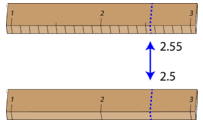# 3.16: Significant Figures

$$\newcommand{\vecs}{\overset { \rightharpoonup} {\mathbf{#1}} }$$ $$\newcommand{\vecd}{\overset{-\!-\!\rightharpoonup}{\vphantom{a}\smash {#1}}}$$$$\newcommand{\id}{\mathrm{id}}$$ $$\newcommand{\Span}{\mathrm{span}}$$ $$\newcommand{\kernel}{\mathrm{null}\,}$$ $$\newcommand{\range}{\mathrm{range}\,}$$ $$\newcommand{\RealPart}{\mathrm{Re}}$$ $$\newcommand{\ImaginaryPart}{\mathrm{Im}}$$ $$\newcommand{\Argument}{\mathrm{Arg}}$$ $$\newcommand{\norm}{\| #1 \|}$$ $$\newcommand{\inner}{\langle #1, #2 \rangle}$$ $$\newcommand{\Span}{\mathrm{span}}$$ $$\newcommand{\id}{\mathrm{id}}$$ $$\newcommand{\Span}{\mathrm{span}}$$ $$\newcommand{\kernel}{\mathrm{null}\,}$$ $$\newcommand{\range}{\mathrm{range}\,}$$ $$\newcommand{\RealPart}{\mathrm{Re}}$$ $$\newcommand{\ImaginaryPart}{\mathrm{Im}}$$ $$\newcommand{\Argument}{\mathrm{Arg}}$$ $$\newcommand{\norm}{\| #1 \|}$$ $$\newcommand{\inner}{\langle #1, #2 \rangle}$$ $$\newcommand{\Span}{\mathrm{span}}$$$$\newcommand{\AA}{\unicode[.8,0]{x212B}}$$Figure $$\PageIndex{1}$$ (Credit: User:WhisperToMe/Wikimedia Commons; Source: http://commons.wikimedia.org/wiki/File:JacintoCitySignJacintoCityTexas.JPG(opens in new window); License: Public Domain)

### How fast do you drive?

As you enter the town of Jacinto City, Texas, a sign tells you that the speed limit is 30 miles per hour. But what if you happen to be driving 31 miles an hour? Are you in trouble? Probably not, because there is a certain amount of leeway built into enforcing the regulation. Most speedometers do not measure the vehicle speed very accurately and could easily be off by a mile or so (on the other hand, radar measurements are much more accurate). So, a couple of miles per hour difference won't matter that much. However, if you stretch the limits any further, you may end up receiving a traffic ticket.

## Significant Figures

The significant figures in a measurement consist of all the certain digits in that measurement, plus one uncertain or estimated digit. In the ruler illustration below, the bottom ruler gave a length with 2 significant figures, while the top ruler gave a length with 3 significant figures. In a correctly reported measurement, the final digit is significant but not certain. Insignificant digits are not reported. With either ruler, it would not be possible to report the length at $$2.553 \: \text{cm}$$, as there is no possible way that the thousandths digit could be estimated. The 3 is not significant and would not be reported.Figure $$\PageIndex{2}$$: Measurement with two different rulers. (Credit: Christopher Auyeung; Source: CK-12 Foundation; License: CC BY-NC-SA 3.0(opens in new window))

When you look at a reported measurement, it is necessary to be able to count the number of significant figures. The table below details the rules for determining the number of significant figures in a reported measurement. For the examples in the table, assume that the quantities are correctly reported values of a measured quantity.

Rule Examples
Figure $$\PageIndex{1}$$: Significant Figure Rules
1. All nonzero digits in a measurement are significant.
• 237 has three significant figures.
• 1.897 has four significant figures.
2. Zeros that appear between other nonzero digits are always significant.
• 39,004 has five significant figures.
• 5.02 has three significant figures.
3. Zeros that appear in front of all of the nonzero digits are called left-end zeros. Left-end zeros are never significant.
• 0.008 has one significant figure.
• 0.000416 has three significant figures.
4. Zeros that appear after all nonzero digits are called right-end zeros. Right-end zeros in a number that lacks a decimal point are not significant.
• 140 has two significant figures.
• 75,210 has four significant figures.
5. Right-end zeros in a number with a decimal point are significant. This is true whether the zeros occur before or after the decimal point.
• 620.0 has four significant figures.
• 19.000 has five significant figures.

It needs to be emphasized that to say a certain digit is not significant, does not mean that it is not important or can be left out. Though the zero in a measurement of 140 may not be significant, the value cannot simply be reported as 14. An insignificant zero functions as a placeholder for the decimal point. When numbers are written in scientific notation, this becomes more apparent. The measurement 140 can be written as $$1.4 \times 10^2$$ with two significant figures in the coefficient. For a number with left-end zeros, such as 0.000416, it can be written as $$4.16 \times 10^{-4}$$ with 3 significant figures. In some cases, scientific notation is the only way to correctly indicate the correct number of significant figures. In order to report a value of 15,000,000 with four significant figures, it would need to be written as $$1.500 \times 10^7$$. The right-end zeros after the 5 are significant. The original number of 15,000,000 only has two significant figures.

## Summary

• Significant figures give an indication of the certainty of a measurement.
• Rules allow decisions to be made about how many digits to use in any given situation.

## Review

1. What does a significant figure tell us?
2. What is a left-end zero?
3. What is a right-end zero?
4. What does an insignificant zero do?

This page titled 3.16: Significant Figures is shared under a CK-12 license and was authored, remixed, and/or curated by CK-12 Foundation via source content that was edited to the style and standards of the LibreTexts platform; a detailed edit history is available upon request.